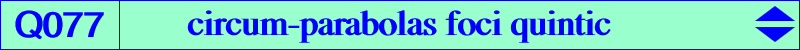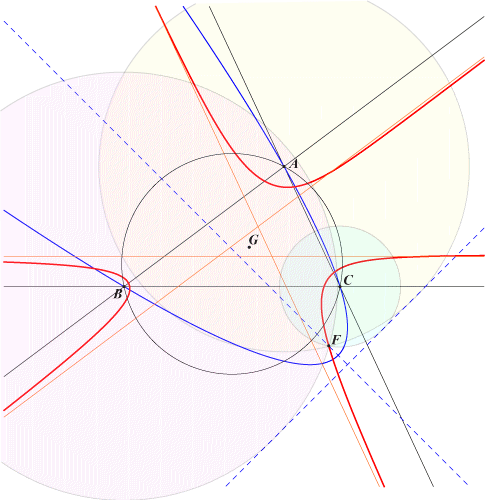too complicated to be written here. Click on the link to download a text file.X(12064), X(38017), X(38018), X(38019), X(38020), X(38233), X(38246) infinite points of the sidelines of ABC common points of (O) and the in/excircles, see belowQ077 is the locus of foci of circum-parabolas. The appearance of (i,j) in the following list means that X(i) is on the line at infinity and X(j) is the corresponding focus of the circumparabola passing through X(i) : (30,38246), (512,38017), (513,38018), (514,38019), (523,12064), (524,38020), (525,38233). Q077 is a circular quintic with three real asymptotes forming a triangle homothetic of ABC under the homothety h(G, 1/4) and two other imaginary asymptotes concurring at X(143), the nine point center of the orthic triangle. It follows that the three circles with centers A, B, C passing through any point F on Q077 must have a common tangent, the directrix of the circum-parabola with focus F. *** Construction of Q077 Let L be a variable line passing through G (or any other point). The complement of the isotomic conjugate of the infinite point Q of L is the perspector P of a circum-parabola. The isogonal conjugate of Q with respect to the anticevian triangle of P is a point F on Q077. *** Locus property (Randy Hutson, private message, 2020-04-28)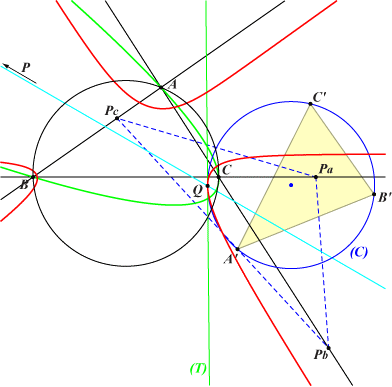Let P be a point on the line at infinity. Let A'B'C' be the orthic triangle of the cevian triangle PaPbPc of P. A'B'C' is perspective to ABC, and the locus of the perspector Q, as P varies, is Q077. Q also lies on the nine-point circle (C) of the cevian triangle of P and (C) is tangent at Q to Q077. See below for a related approach.With the same notations as above, consider the pencil of rectangular circum-hyperbolas of PaPbPc whose centers lie on (C). Then the pole of P in this pencil is F, the focus of the circum-parabola (P) of ABC passing through P. Now, if P' is the infinite point of the direction perpendicular to P, the pole of P in the pencil of circum-conics of PaPbPc passing through P' is the vertex of the parabola above. As such, it must lie on Q079. P' is called the orthopoint of P in ETC hence this vertex is the cross conjugate of P' and P. *** Some additional properties Let G' = P^3, O', H' be the centroid, circumcenter, orthocenter of PaPbPc respectively. gP is the isogonal conjugate of P and cgP is its complement. M is the midpoint of GG'. Then, F verifies these combos : F = gP + 3 G' = cgP - 3 M.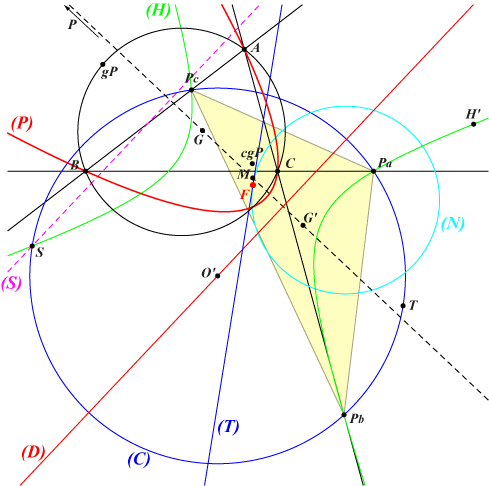Denote by (S) the Simson line of gP in ABC and S the isogonal conjugate in PaPbPc of its infinite point. S obviously lies on the circumcircle (C) of PaPbPc and its antipode on (C) is T. Then F is the common midpoint of SH' and TgP. The directrix (D) of (P) is the parallel at O' to (S). The isogonal transform of (D) in PaPbPc is the rectangular hyperbola (H) passing through Pa, Pb, Pc, H', S and its center is F, clearly on the nine-point circle (N) of PaPbPc. The polar line of P in (H) is (T), the tangent at F to (N) and also the tangent at F to Q077.*** Points of Q077 on the circumcircle (O) of ABC Q077 meets (O) at ten points or, more precisely, five pairs of points and two of these points are the circular points at infinity.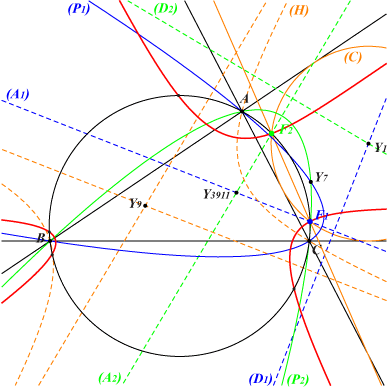Each other pair of points is related to one of the in/excenters as follows. The points related to the incenter X1 are always imaginary and those related to the excenters are always real. The figure opposite is made with the excenter Ib, the b-extraversion of X1 and we shall denote by Yi one of the extraversions of Xi. The inscribed circle with center Y1 meets (O) at F1, F2 which are the foci of two circum-parabolas (P1), (P2) with directrices (D1), (D2) and axes (A1), (A2). Note that (P1), (P2) meet again at Y7. (A1), (A2) are the parallels at Y3911 to the asymptotes of the circum-conic (H) with perspector Y1 and center Y9. (D1), (D2) are the perpendiculars at Y1 to these same asymptotes. The line F1F2 is the radical axis of (O) and (C). It is the trilinear polar of Y279, the barycentric square of Y7.In conclusion, there are exactly six real points on (O) which are the foci of six circum-parabolas of ABC.